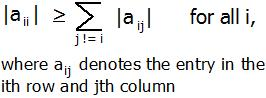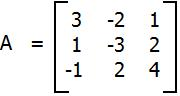# Python Program for Diagonally Dominant Matrix

• Last Updated : 25 May, 2022

In mathematics, a square matrix is said to be diagonally dominant if for every row of the matrix, the magnitude of the diagonal entry in a row is larger than or equal to the sum of the magnitudes of all the other (non-diagonal) entries in that row. More precisely, the matrix A is diagonally dominant ifFor example, The matrixis diagonally dominant because
|a11| ≥ |a12| + |a13| since |+3| ≥ |-2| + |+1|
|a22| ≥ |a21| + |a23| since |-3| ≥ |+1| + |+2|
|a33| ≥ |a31| + |a32| since |+4| ≥ |-1| + |+2|
Given a matrix A of n rows and n columns. The task is to check whether matrix A is diagonally dominant or not.
Examples :

```Input : A = { { 3, -2, 1 },
{ 1, -3, 2 },
{ -1, 2, 4 } };
Output : YES
Given matrix is diagonally dominant
because absolute value of every diagonal
element is more than sum of absolute values
of corresponding row.

Input : A = { { -2, 2, 1 },
{ 1, 3, 2 },
{ 1, -2, 0 } };
Output : NO```

The idea is to run a loop from i = 0 to n-1 for the number of rows and for each row, run a loop j = 0 to n-1 find the sum of non-diagonal element i.e i != j. And check if diagonal element is greater than or equal to sum. If for any row, it is false, then return false or print “No”. Else print “YES”.

## Python3

 `# Python Program to check``# whether given matrix is``# Diagonally Dominant Matrix.` `# check the given ``# matrix is Diagonally``# Dominant Matrix or not.``def` `isDDM(m, n) :` `    ``# for each row``    ``for` `i ``in` `range``(``0``, n) :        ``    ` `        ``# for each column, finding``        ``# sum of each row.``        ``sum` `=` `0``        ``for` `j ``in` `range``(``0``, n) :``            ``sum` `=` `sum` `+` `abs``(m[i][j])    ` `        ``# removing the``        ``# diagonal element.``        ``sum` `=` `sum` `-` `abs``(m[i][i])` `        ``# checking if diagonal``        ``# element is less than``        ``# sum of non-diagonal``        ``# element.``        ``if` `(``abs``(m[i][i]) < ``sum``) :``            ``return` `False` `    ``return` `True` `# Driver Code``n ``=` `3``m ``=` `[[ ``3``, ``-``2``, ``1` `],``    ``[ ``1``, ``-``3``, ``2` `],``    ``[ ``-``1``, ``2``, ``4` `]]` `if``((isDDM(m, n))) :``    ``print` `(``"YES"``)``else` `:``    ``print` `(``"NO"``)` `# This code is contributed by``# Manish Shaw(manishshaw1)`

Output :

`YES`

Time Complexity: O(N2)

Auxiliary Space: O(1)

Please refer complete article on Diagonally Dominant Matrix for more details!

My Personal Notes arrow_drop_up